Latest Banking jobs   »   Quantitative Aptitude Quiz For SBI PO...

# Quantitative Aptitude Quiz For SBI PO Prelims 2022- 29th November

Direction (1 – 5): In the given questions, two quantities are given one as ‘Quantity I’ and another as ‘Quantity II’. You have to determine relationship between two quantities and choose the appropriate option.

Q1. Three numbers A, B and C in the ratio of 25 : 22 : 13 respectively and their average is 300.
Quantity I – If A increased by 20% , B increased by 15%, then find C increased by what percent, if their average increased by 16 ⅔%.
Quantity II – 13%
(a) Quantity I > Quantity II
(b) Quantity I < Quantity II
(c) Quantity I ≥ Quantity II
(d) Quantity I = Quantity II or no relation
(e) Quantity I≤ Quantity II

Q2. Average of present ages of Anurag, Bhavesh and Chindi is 35 years. One year ago, Bhavesh is twice as old as Chindi, while five years hence, Anurag is two-third as old as Bhavesh.
Quantity I – Find the age of Anurag, when Chindi was born.
Quantity II – 6 years
(a) Quantity I > Quantity II
(b) Quantity I < Quantity II
(c) Quantity I ≥ Quantity II
(d) Quantity I = Quantity II or no relation
(e) Quantity I≤ Quantity II

Q3. Quantity I:– A square of side 7/2 meter is bent into form of a circle. Area of circle.
Quantity II:– A cylinder of height 7 meter and radius of base is 50-centimeter curved surface area of cylinder is.
(a) Quantity I > Quantity II
(b) Quantity I < Quantity II
(c) Quantity I ≥ Quantity II
(d) Quantity I = Quantity II or no relation
(e) Quantity I≤ Quantity II

Q4. 480 meters & 420 meters long train running in opposite direction cross each other in 12 sec. Ratio between speed of longer train and smaller train is 7 : 8 and faster train cross a platform in 18 sec.
Quantity I – Time taken (in sec) by slower train to cross a bridge, which is 120 meters longer than platform.
Quantity II – 26 sec
(a) Quantity I > Quantity II
(b) Quantity I < Quantity II
(c) Quantity I ≥ Quantity II
(d) Quantity I = Quantity II or no relation
(e) Quantity I≤ Quantity II

Q5. A boat can cover 5 km distance in 30 minutes in still water. Speed of stream is half of speed of boat in still water.
Quantity I – If boat takes 2⅔ hour to row a place and back, then find how far is the place.
Quantity II – 8750 meters
(a) Quantity I > Quantity II
(b) Quantity I < Quantity II
(c) Quantity I ≥ Quantity II
(d) Quantity I = Quantity II or no relation
(e) Quantity I≤ Quantity II

Directions (6-10):- Each of the following question is followed by two quantities I, and II. You have to determine the value of the quantities using the information provided and compare the quantities to answer as per the instruction set provided below.
(a) Quantity I>Quantity II
(b) Quantity I≥Quantity II
(c) Quantity I<Quantity II
(d) Quantity I≤Quantity II
(e) Quantity I=Quantity II or no relation

Q6. What is the number?
Quantity I: 70% of a number is 63.
Quantity II: 20% of number is 40.

Q7. Find the distance covered.
Quantity I: the speed of bike is 20 kmph throughout the journey of 2 hours.
Quantity II: it takes 3 hours to cover a distance at uniform speed of 12 kmph.

Q8. Find the population of town in previous year.
Quantity I: the population increases every year by 10% and it is 11000 at present.
Quantity II: in previous year, male population was 40% more than female population which was 5000.

Q9.
Quantity I: average marks in physics & chemistry is 75 while that in physics, chemistry & maths is 80. Find marks in mathematics.
Quantity II: 90

Q10.
Quantity I: Raj spends 30% of his income on rent and 40% of remaining on miscellaneous. If he saves Rs. 630. Find his income.
Quantity II: Simran’s present monthly salary is Rs. 10000 of which she spends 70% on rent, food & clothes. If she saves Rs. 1500. What can be her expenditure on travel?

Directions (11-15): The following questions are accompanied by two statements (I) and (II). You have to determine which statements(s) is/are sufficient/necessary to answer the questions.
(a) Statement I alone is sufficient to answer the question but statement II alone is not sufficient to answer the question.
(b) Statement II alone is sufficient to answer the question but statement I alone is not sufficient to answer the question.
(c) Both the statements taken together are necessary to answer the question, but neither of the statements alone is sufficient to answer the question.
(d) Either statement I or statement II by itself is sufficient to answer the question.
(e) Statements I and II taken together are not sufficient to answer the question.

Q11. What is the marked price of the article?
(I) A shopkeeper marks his article 60% above the cost price and he allows 6 ¼ % discount on marked price and ratio of cost price to selling price of article is 2 : 3.
(II) Shopkeeper earns Rs.60 as profit on selling the article.

Q12. Find in how many days A alone can complete the work.
(I) B and D working together can complete a piece of work in 20 days. A is 25% less efficient than B and A & D working together can complete same piece of work in 22.5 days.
(II) C can complete a piece of work in 50 days and C’s efficiency is 120% of A’s efficiency.

Q13. Find ratio of b to c.
(I) Sum of b & c is 8 and difference between squares of a and c is zero.
(II)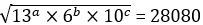Q14. Find ratio of speed of boat in still water to speed of stream.
(I) Ratio of time taken by boat to cover 40 km in upstream to 40 km in downstream is 5 : 1.
(II) Time taken by boat to cover 80 km in downstream is 8 hours less than the time taken by boat to cover 48 km in upstream.

Q15. What will be the 15th term of an A.P.?
(I) 6th term of an A.P. is 8 times of the first term and sum of first 5 terms of an A.P. is 95.
(II) Ratio of 5th to 8th term of an A.P. is 11 : 18.

Solutions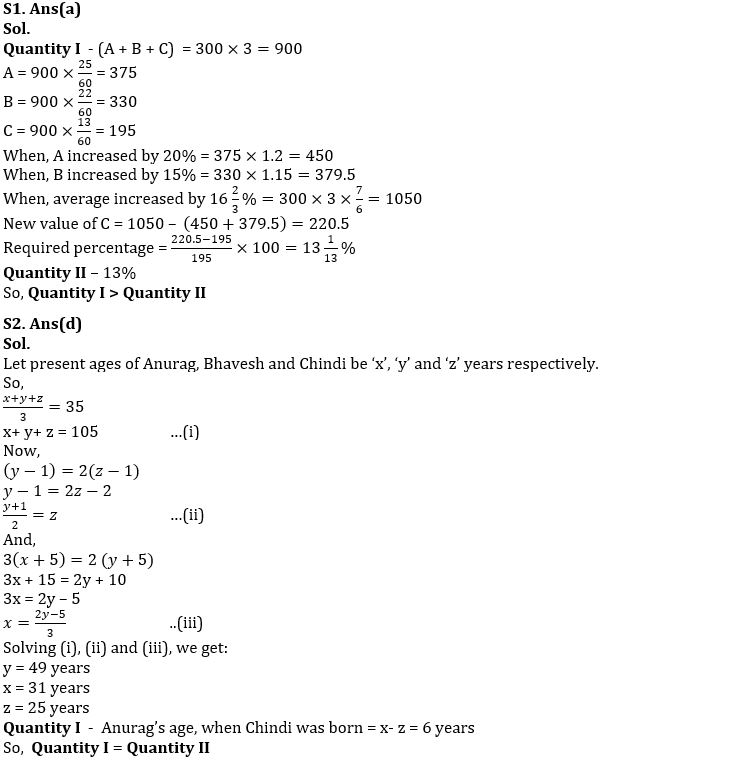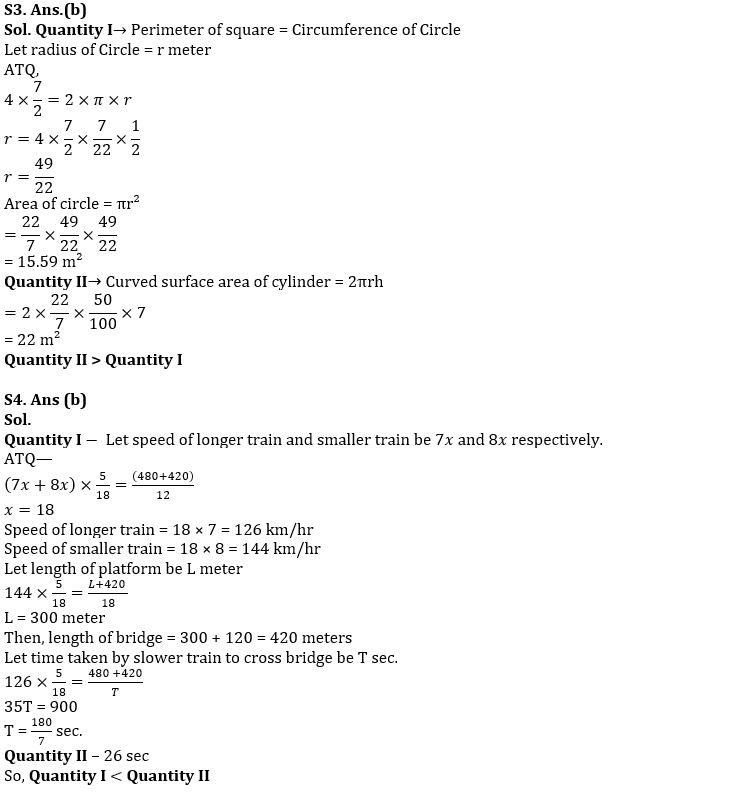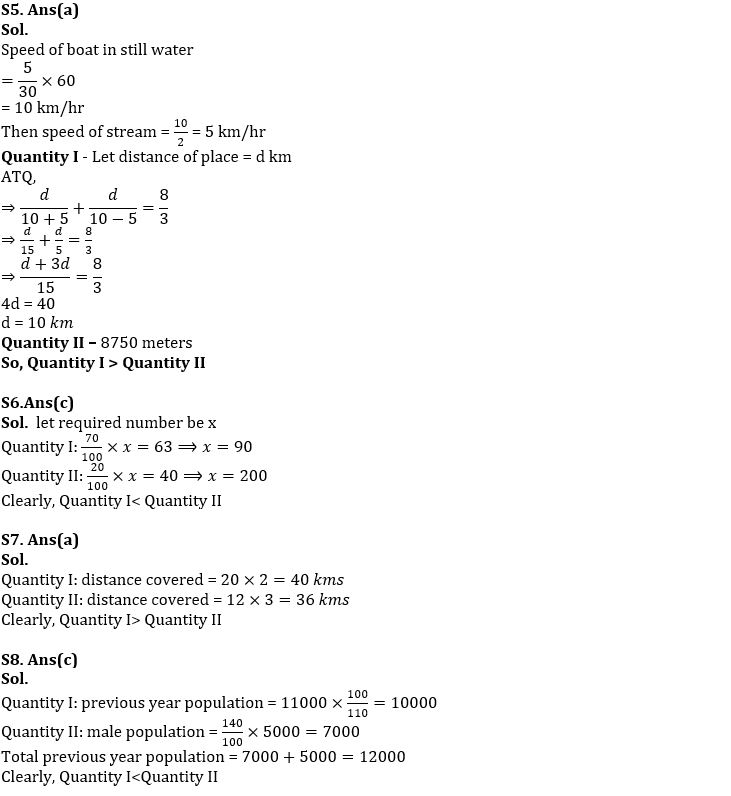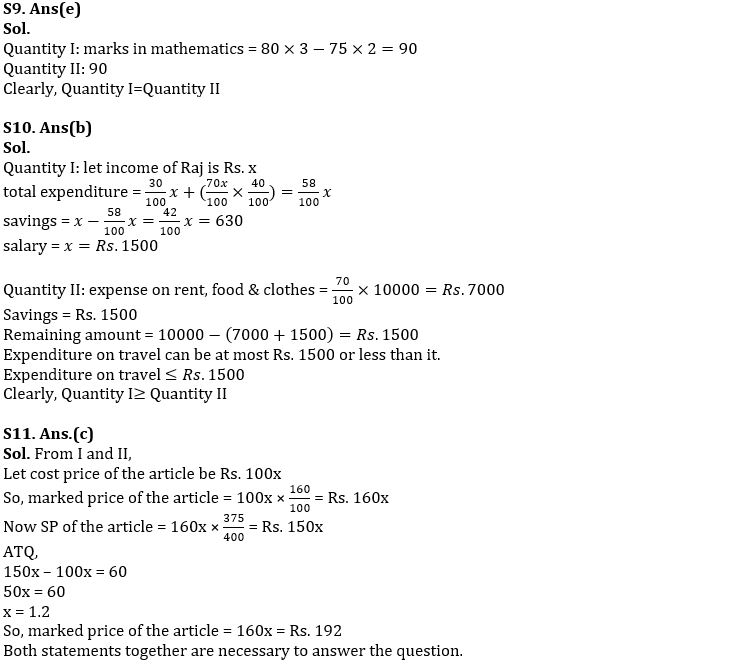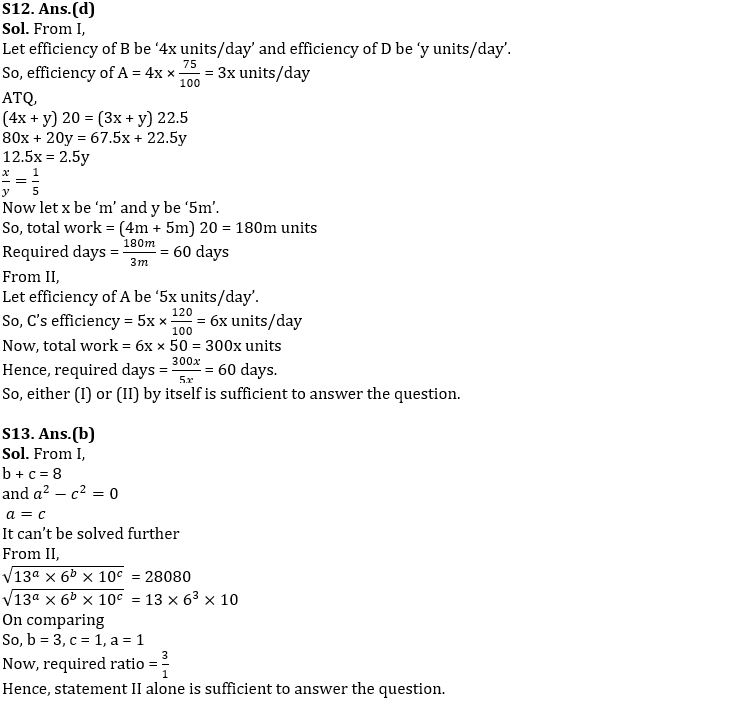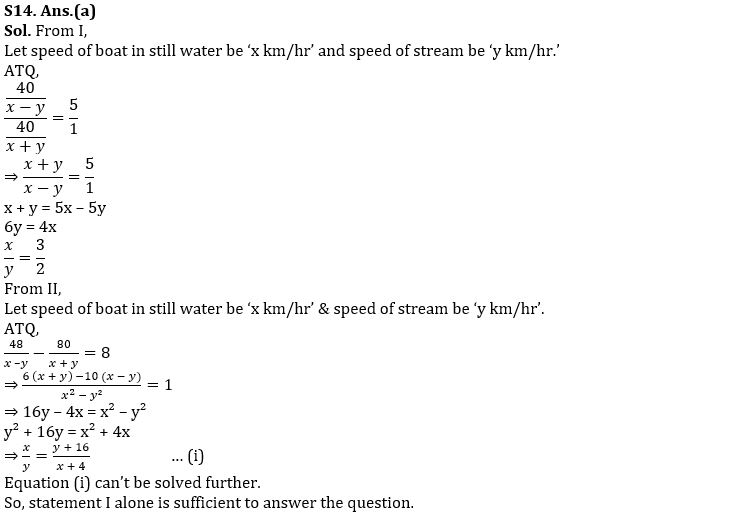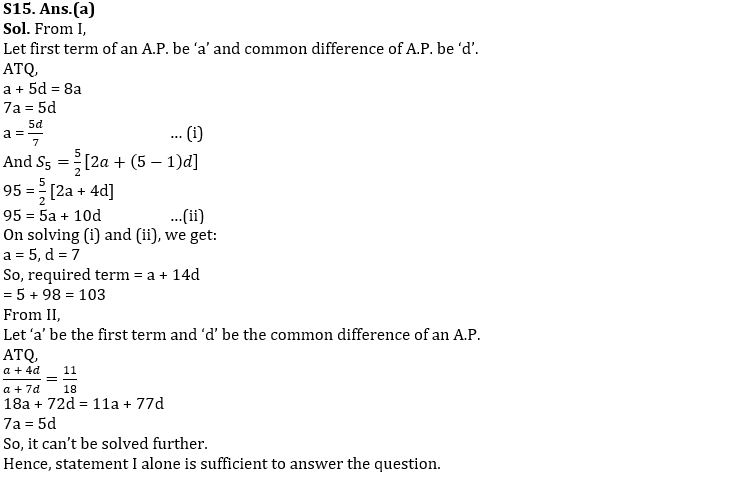#### Congratulations!Union Budget 2023-24: Free PDF